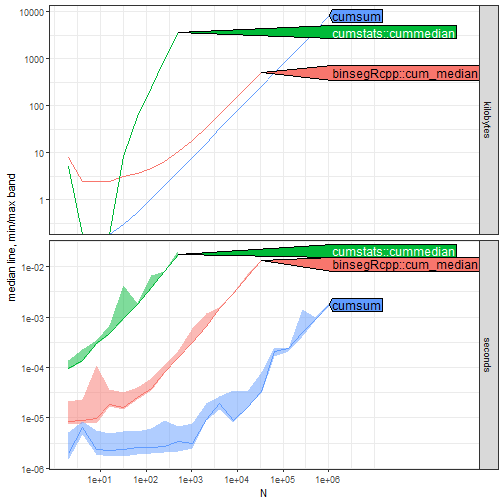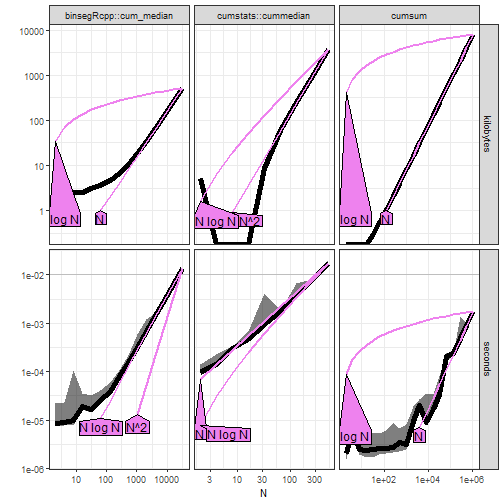# Cumulative median

In this vignette we compare two different implementations of the cumulative median. The cumstats package provides a naive method, which uses the standard median function in a for loop. Each call to the standard median function is log-linear, so the total expected complexity is log-quadratic. The binsegRcpp package provides a different implementation that uses a log-linear algorithm, previously described in the 2017 NeurIPS research paper Maximum Margin Interval Trees by Alexandre Drouin, Toby Hocking, Francois Laviolette.

``````expr.list <- c(
if(requireNamespace("cumstats"))atime::atime_grid(
"cumstats::cummedian"=cumstats::cummedian(data.vec)),
if(requireNamespace("binsegRcpp"))atime::atime_grid(
"binsegRcpp::cum_median"=binsegRcpp::cum_median(data.vec)),
atime::atime_grid(cumsum=cumsum(data.vec)))
atime.list <- atime::atime(
N=2^seq(1, 20),
setup={
set.seed(1)
data.vec <- rnorm(N)
},
result=TRUE,
expr.list=expr.list,
times=5)
plot(atime.list)
#> Warning: Transformation introduced infinite values in continuous y-axis
#> Transformation introduced infinite values in continuous y-axis
#> Transformation introduced infinite values in continuous y-axis
````````````(best.list <- atime::references_best(atime.list))
#> references_best list with 88 measurements, best fit complexity:
#> cumstats::cummedian (N^2 kilobytes, N log N seconds)
#> binsegRcpp::cum_median (N kilobytes, N log N seconds)
#> cumsum (N kilobytes, N seconds)
## try() to avoid CRAN error 'from' must be a finite number, onExercise for the reader: increase `seconds.limit` and max `N` until you can clearly show that `binsegRcpp::cum_median` should be the preferred method for computing the cumulative median.